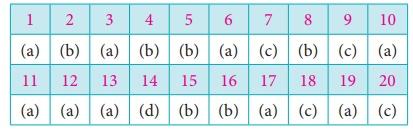Home | | Business Maths 12th Std | Choose the correct Answer

## Chapter: 12th Business Maths and Statistics : Chapter 8 : Sampling Techniques and Statistical Inference

Maths: Sampling Techniques and Statistical Inference: Multiple choice questions with Answers, Solution - Book back 1 mark questions and answers with solution for Exercise Problems

1. A …………. may be finite or infinite according as the number of observations or items in it is finite or infinite.

(a) Population

(b) census

(c) parameter

(d) none of these

2. A ……… of statistical individuals in a population is called a sample.

(a) Infinite set

(b) finite subset

(c) finite set

(d) entire set

3. A finite subset of statistical individuals in a population is called ………...

(a) a sample

(b) a population

(c) universe

(d) census

4. Any statistical measure computed from sample data is known as …………...

(a) parameter measure

(b) statistic

(c) infinite measure

(d) uncountable

5. A…………..is one where each item in the universe has an equal chance of known opportunity of being selected.

(a) Parameter

(b) random sample

(c) statistic

(d) entire data

6. A random sample is a sample selected in such a way that every item in the population has an equal chance of being included

(a) Harper

(b) Fisher

(c) Karl Pearson

(d) Dr. Yates

7. Which one of the following is probability sampling

(a) purposive sampling

(b) judgment sampling

(c) simple random sampling

(d) Convenience sampling

8. In simple random sampling from a population of units, the probability of drawing any unit at the first draw is

(a) n/N

(b) 1/N

(c) N/n

(d) 1

9. In …………….. the heterogeneous groups are divided into homogeneous groups.

(a) Non-probability sample

(b) a simple random sample

(c) a stratified random sample

(d) systematic random sample

10. Errors in sampling are of

(a) Two types

(b) three types

(c) four types

(d) five types

11. The method of obtaining the most likely value of the population parameter using statistic is called ……………………

(a) estimation

(b) estimator

(c) biased estimate

(d) standard error.

12. An estimator is a sample statistic used to estimate a

(a) population parameter

(b) biased estimate

(c) sample size

(d) census

13.  …………..is a relative property, which states that one estimator is efficient relative to another.

(a) efficiency

(b) sufficiency

(c) unbiased

(d) consistency

14. If probability P[| θ − θ |< ε] → 1µ as n → ∞′ , for any positive ε then θ is said to …………..estimator of θ .

(a) efficient

(b) sufficient

(c) unbiased

(d) consistent

15. An estimator is said to be …………. if it contains all the information in the data about the parameter it estimates.

(a) efficient

(b) sufficient

(c) unbiased

(d) consistent

16. An estimate of a population parameter given by two numbers between which the parameter would be expected to lie is called an……………..interval estimate of the parameter.

(a)point estimate

(b) interval estimation

(c) standard error

(d) confidence

17. A ________ is a statement or an assertion about the population parameter.

(a) hypothesis

(b) statistic

(c) sample

(d) census

18. Type I error is

(a) Accept H0 when it is true

(b) Accept H0 when it is false

(c) Reject H0 when it is true

(d) Reject H0 when it is false.

19. Type II error is

(a) Accept H0 when it is wrong

(b) Accept H0 when it is true

(c) Reject H0 when it is true

(d) Reject H0 when it is false

20. The standard error of sample mean is

(a) σ /√2n

(b) σ /n

(c) σ /√n

(d) s2/√nTags : Sampling Techniques and Statistical Inference , 12th Business Maths and Statistics : Chapter 8 : Sampling Techniques and Statistical Inference
Study Material, Lecturing Notes, Assignment, Reference, Wiki description explanation, brief detail
12th Business Maths and Statistics : Chapter 8 : Sampling Techniques and Statistical Inference : Choose the correct Answer | Sampling Techniques and Statistical Inference